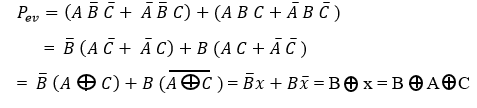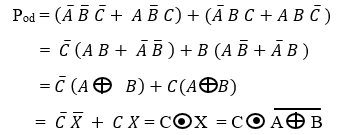# What is Parity Generator and Parity Checker : Types & Its Logic Diagrams

The parity generator and parity checker’s main function is to detect errors in data transmission and this concept is introduced in 1922. In RAID technology the parity bit and the parity checker are used to guard against data loss. The parity bit is an extra bit that is set at the transmission side to either ‘0’ or ‘1’, it is used to detect only single bit error and it is the easiest method for detecting errors. There are different types of error detection codes used to detect the errors they are parity, ring counter, block parity code, Hamming code, biquinary, etc. The brief explanation about parity bit, parity generator and checker are explained below.

## What is Parity Bit?

Definition: The parity bit or check bit are the bits added to the binary code to check whether the particular code is in parity or not, for example, whether the code is in even parity or odd parity is checked by this check bit or parity bit. The parity is nothing but number of 1’s and there are two types of parity bits they are even bit and odd bit.

In odd parity bit, the code must be in an odd number of 1’s, for example, we are taking 5-bit code 100011, this code is said to be odd parity because there is three number of 1’s in the code which we have taken. In even parity bit the code must be in even number of 1’s, for example, we are taking 6-bit code 101101, this code is said to be even parity because there are four number of 1’s in the code which we have taken

## What is the Parity Generator?

Definition: The parity generator is a combination circuit at the transmitter, it takes an original message as input and generates the parity bit for that message and the transmitter in this generator transmits messages along with its parity bit.

### Types of Parity Generator

The classification of this generator is shown in the below figure

### Even Parity Generator

The even parity generator maintains the binary data in even number of 1’s, for example, the data taken is in odd number of 1’s, this even parity generator is going to maintain the data as even number of 1’s by adding the extra 1 to the odd number of 1’s. This is also a combinational circuit whose output is dependent upon the given input data, which means the input data is binary data or binary code given for parity generator.

Let us consider three input binary data, that three bits are considered as A, B, and C. We can write 23 combinations using the three input binary data that is from 000 to 111 (0 to 7), total eight combinations will get from the given three input binary data which we have considered. The truth table of even parity generator for three input binary data is shown below.

0 0 0 – In this input binary code the even parity is taken as ‘0’ because the input is already in even parity, so no need to add even parity once again for this input.

0 0 1 – – In this input binary code there is only a single number of ‘1’ and that single number of ‘1’ is an odd number of ‘1’. If an odd number of ‘1’ is there, then even parity generator must generate another ‘1’ to make it as even parity, so even parity is taken as 1 to make the 0 0 1 code into even parity.

0 1 0 –  This bit is in odd parity so even parity is taken as 1 to make the 0 1 0 code into even parity.

0 1 1 – This bit is already in even parity so even parity is taken as 0 to make the 0 1 1 code into even parity.

1 0 0 – This bit is in odd parity so even parity is taken as 1 to make the 1 0 0 code into even parity.

1 0 1 – This bit is already in even parity so even parity is taken as 0 to make the 1 0 1 code into even parity.

1 1 0 – This bit is also in even parity so even parity is taken as 0 to make the 1 1 0 code into even parity.

1 1 1 – This bit is in odd parity so even parity is taken as 1 to make the 1 1 1 code into even parity.

#### Even Parity Generator Truth Table

 A B C Even Parity 0  0 0 0 0  0 1 1 0  1 0 1 0  1 1 0 1  0 0 1 1  0 1 0 1  1 0 0 1  1 1 1

The karnaugh map (k-map) simplification for three-bit input even parity is

From the above even parity truth table, the parity bit simplified expression is written asThe even parity expression implemented by using two Ex-OR gates and the logic diagram of this even parity using the Ex-OR logic gate is shown below.

In this way, the even parity generator generates an even number of 1’s by taking the input data.

### Odd Parity Generator

The odd parity generator maintains the binary data in an odd number of 1’s, for example, the data taken is in even number of 1’s, this odd parity generator is going to maintain the data as an odd number of 1’s by adding the extra 1 to the even number of 1’s. This is the combinational circuit whose output is always dependent upon the given input data.  If there is an even number of 1’s then only parity bit is added to make the binary code into an odd number of 1’s.

Let us consider three input binary data, that three bits are considered as A, B, and C. The truth table of odd parity generator for three input binary data is shown below.

0 0 0 – In this input binary code the odd parity is taken as ‘1’ because the input is in even parity.

0 0 1 –  This binary input is already in odd parity, so odd parity is taken as 0.

0 1 0 – This binary input is also in odd parity, so odd parity is taken as 0.

0 1 1 – This bit is in even parity so odd parity is taken as 1 to make the 0 1 1 code into odd parity.

1 0 0 – This bit is already in odd parity, so odd parity is taken as 0 to make the 1 0 0 code into odd parity.

1 0 1 – This input bit is in even parity, so odd parity is taken as 1 to make the 1 0 1 code into odd parity.

1 1 0 – This bit is in even parity, so odd parity is taken as 1.

1 1 1 – This input bit is in odd parity, so odd parity is taken as o.

#### Odd Parity Generator Truth Table

 A B C Odd Parity 0  0 0 1 0  0 1 0 0  1 0 0 0  1 1 1 1  0 0 0 1  0 1 1 1  1 0 1 1  1 1 0

The Kavanaugh map (k-map) simplification for three-bit input odd parity is

From the above odd parity truth table, the parity bit simplified expression is written asThe logic diagram of this odd parity generator is shown below.

In this way, the odd parity generator generates an odd number of 1’s by taking the input data.

## What is the Parity Check?

Definition: The combinational circuit at the receiver is the parity checker. This checker takes the received message including the parity bit as input. It gives output ‘1’ if there is some error found and gives output ‘0’ if no error is found in the message including the parity bit.

### Types of Parity Checker

The classification of the parity checker is shown in the below figure

### Even Parity Checker

In even parity checker if the error bit (E) is equal to ‘1’, then we have an error. If error bit E=0 then indicates there is no error.

Error Bit (E) =1, error occurs

Error Bit (E) =0, no error

The parity checker circuit is shown in the below figure

### Odd Parity Checker

In odd parity checker if an error bit (E) is equal to ‘1’, then it indicates there is no error. If an error bit E=0 then indicates there is an error.

Error Bit (E) =1, no error

Error Bit (E) =0, error occurs

The parity checker won’t be able to detect if there are errors in more than ‘1’ bit and the correct of data is also not possible, these are the main disadvantages of the parity checker.

### Parity Generator/Checker using IC’s

The IC 74180 does the function of parity generation as well as checking. The 9 bit (8 data bits, 1 parity bit) Parity Generator/Checker is shown in the below figure.

The IC 74180 contains eight data bits (X0 to X7), Vcc, even input, odd input, Seven output, S odd output, and ground pin.

If the given even and odd input both are high (H), then the even and odd outputs both are low (L), similarly, if the given inputs both are Low (L), then the even and odd outputs both becomes high (H).

• Simplicity
• Easy to use

### Applications of Parity

The applications of parity are

• In digital systems and many hardware applications, this parity is used
• The parity bit is also used in Small Computer System Interface (SCSI) and also in Peripheral Component Interconnect (PCI) to detect the errors

### FAQs

1). What is the difference between the parity generator and parity checker?

The parity generator generates the parity bit in the transmitter and the parity checker checks the parity bit in the receiver.

2). What does no parity mean?

When the parity bits are not used to check for errors then the parity bit is said to be non-parity or no parity or the absence of parity.

3). What is the parity value?

The parity value concept used for both commodities and securities and the term refers to when the value of the two assets is equal.

4). Why do we need a parity checker?

The parity checker is needed to detect the errors in communication and also in the memory storage devices parity checker is used for testing.

5). How can the parity bit detect a damaged data unit?

The redundant bit in this technique is called a parity bit, it detects damaged data unit when an error occurs during the transmission of data.

In this article, how the parity generator and checker generate and check the bit and its types, logic circuits, truth tables, and k-map expressions are discussed briefly. Here is a question for you, how do you calculate even and odd parity?